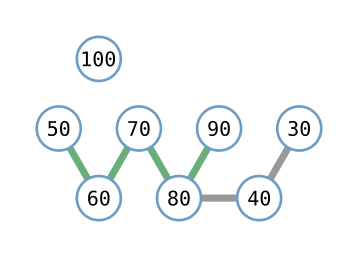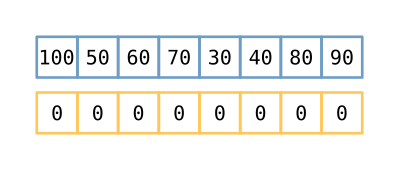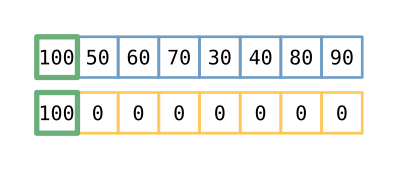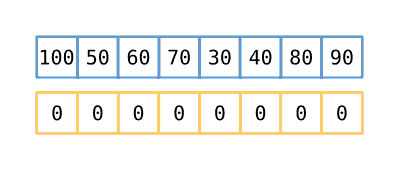# Longest Increasing Subsequence

By Eric Burden | November 8, 2021

Photo by Allan Mas from Pexels

## The Problem

Recently, I was posed the “Longest Increasing Subsequence” problem in the CodeConnector community on Slack. It’s one of those coding questions that shows up in some form or another on a lot of coding puzzle/interview prep sites. If you haven’t seen it before (like I hadn’t), the problem statement is as follows (this description comes from LeetCode):

Given an integer array nums, return the length of the longest strictly increasing subsequence.

A subsequence is a sequence that can be derived from an array by deleting some or no elements without changing the order of the remaining elements. For example, [3,6,2,7] is a subsequence of the array [0,3,1,6,2,2,7].

Example 1:

Input: nums = [10,9,2,5,3,7,101,18]
Output: 4
Explanation: The longest increasing subsequence is [2,3,7,101], therefore the length is 4.


Example 2:

Input: nums = [0,1,0,3,2,3]
Output: 4


Example 3:

Input: nums = [7,7,7,7,7,7,7]
Output: 1


Constraints:

• 1 <= nums.length <= 2500
• -104 <= nums[i] <= 104

Follow up: Can you come up with an algorithm that runs in O(n log(n)) time complexity?

I’ve been on a bit of a graph algorithm kick lately, so the first thing that stood out to me was that this problem could be represented like so:Given an array of [100, 50, 60, 70, 30, 40, 80, 90], we can see that 100 is disconnected from the rest of the ‘graph’ because it is not followed by any larger numbers, there is a sequence 50 -> 60 -> 70 -> 80 -> 90 and a sequence 30 -> 40 -> 80 -> 90, and the longest sequence is length 5.

I won’t dive into the code for this approach, though, because while I was able to get a couple of different implementations using this same idea, none of them could reach that elusive O(n log n) time complexity. The graph approach(es) can come in under O(n^2^) time complexity, but it’s still exponential. (Check out this article if you’d like a primer on Big-O notation).

## The Solution?

As any good programmer does, once I’d exhausted my own creativity, I turned to the internet. One quick search later, and I found a number of articles, including Wikipedia, describing a strategy for solving this problem in O(n log n) time. Unfortunately, they were often not very understandable and generally represented an very faithful reproduction of this pseudocode (from Wikipedia):

P = array of length N
M = array of length N + 1

L = 0
for i in range 0 to N-1:
// Binary search for the largest positive j ≤ L
// such that X[M[j]] < X[i]
lo = 1
hi = L + 1
while lo < hi:
mid = lo + floor((hi-lo)/2)
if X[M[mid]] < X[i]:
lo = mid+1
else:
hi = mid

// After searching, lo is 1 greater than the
// length of the longest prefix of X[i]
newL = lo

// The predecessor of X[i] is the last index of
// the subsequence of length newL-1
P[i] = M[newL-1]
M[newL] = i

if newL > L:
// If we found a subsequence longer than any we've
// found yet, update L
L = newL

// Reconstruct the longest increasing subsequence
S = array of length L
k = M[L]
for i in range L-1 to 0:
S[i] = X[k]
k = P[k]

return S


## My Solution

I’m sure that’s very correct and all, but it’s also a bit tough for me to wrap my head around as written. With a bit of tinkering, I was able to reproduce the same effect using this code in Rust:

#![feature(result_into_ok_or_err)]
fn longest_increasing_sequence(numbers: &[usize]) -> usize {
let mut subsequence = vec![0; numbers.len()];
let mut len = 0;

for number in numbers {
let insertion_point = subsequence[0..len]
.binary_search(number)
.into_ok_or_err();
if subsequence[insertion_point] == 0 { len += 1; }
subsequence[insertion_point] = *number;
}

len
}


If you’re familiar with Rust at all, then it’s probably pretty clear to you what this code is doing, if not exactly why. Let me explain.

### Step 1

Create a vector (array, list, whatever) the same length as the input and fill it with zeros. You may need all of it in the worst case, otherwise there will be some trailing zeros left over. We also start by assuming our longest sequence will be length 0, though that will change quickly. We’re going to put some numbers into this subsequence array, which may or may not end up being our actual subsequence numbers, but will be the same length as our longest subsequence (more on that soon). We also set len to point at the first index. For the rest of this algorithm, len will refer to the index of the first 0 in subsequence, from the left.### Step 2

Take the first number from the input array and place it into the first space in the subsequence array that will not change the order of the numbers already there, not considering the zeros. For this first pass, that means in the first space. Now, because these non-zero numbers will be placed into subsequence sorted, you can find the best space to put the number using a binary search, which has a time complexity of O(log n).

A note about the Rust binary_search() function. binary_search() is a member function for a sorted slice, or view, of an Array or Vector (or String). Given a value, binary_search() will search that sorted slice and return a Result<usize, usize>, which is either Ok(usize) containing the index of the found value, or Err(usize) containing the index where that value could be placed without disrupting the sorted nature of the slice. Since either of those is good for this use case (there’s no harm in replacing a number with itself here), we use into_ok_or_err() to return whichever number is returned in the Result<usize, usize>. The documentation for into_ok_or_err() explicitly calls out this use as one of the reasons for this function to exist.### Step 3

We then proceed down the input slice, placing the number we find into the subsequence array into whatever position will leave all the non-zeros in subsequence sorted. If that position is at the end of the non-zero numbers (e.g. in the position marked by len), then move len one index to the right. You may note that, at some point, the subsequence is no longer in order based on the input array, but it remains sorted. When we’re done placing numbers from the input, the number of non-zero values in subsequence will represent the length of the longest increasing subsequence. Since we’re performing this operation for each item in the input array, this ‘outer’ operation is on the order of O(n), and since we perform a binary search on each iteration, we arrive at our final time complexity of O(n log n).### Step 4

Return len, which indicates both the index of the left-most 0 and the length of the longest increasing subsequence. (They’re the same.)

### Step 5 (Optional)

There are some real-world optimizations that can be added to the code as written above. Most obviously, it’s pretty easy to tell if a new number should replace the first item in subsequence (number is smaller than subsequence) or be inserted into the space of the leftmost zero (number is larger than subsequence[len]). A couple of if statements to that effect could have a noticeable improvement on randomized workloads, allowing you to skip a fair number of the binary search operations. I’d probably also skip inserting number into subsequence if it’s already there, ending up with a (more) final version that looks like:

fn longest_increasing_sequence(numbers: &[usize]) -> usize {
let mut subsequence = vec![0; numbers.len()];
let mut len = 0;

for number in numbers {
// Can avoid a binary search if number is smaller than subsequence
if number <= &subsequence {
subsequence = *number;
continue;
}

// Can avoid a binary search if number is larger than the last non-zero
// value in subsequence. Should also do this on the first iteration to
// place an initial number into subsequence
if len == 0 || number > &subsequence[len - 1] {
subsequence[len] = *number;
len += 1;
continue;
}

// If number is already in subsequence, we can just keep going.
match subsequence[0..len].binary_search(number) {
Ok(_) => continue,
Err(idx) => subsequence[idx] = *number,
}
}

len
}


I think this final version is still very clear and readable, but now I’m doing more things, which warrants some explanatory comments (focused, of course, on why, not what or how). In terms of understanding how this algorithm works, though, this version can obfuscate the core of our process a bit. In terms of real-world run time, this can be quite a bit faster.

## Final Thoughts

One thing that annoys me with pseudocode I find on Wikipedia or real code I find in blog posts is this tendency to ‘budget’ characters. (I’m looking at you, X[k]). I’m not sure if it’s because the individuals writing these posts came to understand the problem so well in the process of preparing the post that they don’t see a need to write more expressive code or if there’s some global perception that more concise code lends credibility to the author or there’s some other reason I have no insight into. Whatever the reason, I would urge authors to consider their audience or run their text by a less-invested third party for a clarity check ahead of time. I can almost guarantee that if your code/pseudocode has single capital letter variable names, it will be inaccessible to at least some folks who could really benefit from the knowledge you’re trying to share. Code written for others in instructional materials should be more (if not much more) readable than production code. I don’t mean to imply that there aren’t clear, well-crafted, super-educational authors and sites out there. For this problem, at least, those really well-written explanations just didn’t show up in my searches. That’s two skills I can work on, I suppose!

If you have any thoughts, comments, or even constructive criticism, please let me know. I’m always looking for places to improve, and I’d to have you join me!